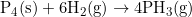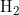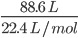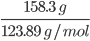×
Get Full Access to Introductory Chemistry - 5 Edition - Chapter 11 - Problem 114p
Get Full Access to Introductory Chemistry - 5 Edition - Chapter 11 - Problem 114p

×

# Consider the reaction:P4(s) + 6H2(g) ? 4 PH3(g)(a) If 88.6 L of H2(g), measured at STPISBN: 9780321910295 34

## Solution for problem 114P Chapter 11

Introductory Chemistry | 5th Edition

• Textbook Solutions
• 2901 Step-by-step solutions solved by professors and subject experts
• Get 24/7 help from StudySoup virtual teaching assistantsIntroductory Chemistry | 5th Edition

4 5 1 240 Reviews
14
5
Problem 114P

Consider the reaction:

$$P_{4}(s)+6\ \mathrm{H}_{2}(g) \rightarrow 4\ \mathrm{PH}_{3}(g)$$

(a) If 88.6 L of $${H}_{2}$$( g), measured at STP, is allowed to react with 158.3 g of $${P}_{4}$$, what is the limiting reactant?

(b) If 48.3 L of $${P H}_{3}$$, measured at STP, forms, what is the percent yield?

Equation Transcription:Text Transcription:

P_4 (s) + 6 H_2 (g) right arrow 4 PH_3 (g)

Step-by-Step Solution:

Step 1 of 4

Given reaction:(a) If 88.6 L of(g), measured at STP, is allowed to react with 158.3 g of, what is the limiting reactant?

To find the limiting reactant, we should know the moles of each reactant. So let’s find the number of moles of each reactant :

First, let’s find number of moles of:

We know, at STP(Standard Temperature and Pressure), one mole of any gas occupies 22.4 L.

Moles of== 3.955 moles.

Now, let’s find number of moles of:

Molar mass of= 123.89 g/mol.

moles of== 1.277 moles.

Step 2 of 4

Step 3 of 4

##### ISBN: 9780321910295

Unlock Textbook Solution